Kite Follow-up Discussion by Art Mabbott << Photos >> Files from Art to download: kites.lesson.doc | tetrahedron.kite.model.doc | kite.worksheets.xls | kites.writeup.doc When building the tetrahedron kites there is so much mathematics embedded in the kites that makes it a valuable excursion. As we start to construct the units, I like to look at the rules for lengths/perimeter, area, and volume. As shown in the chart below, we will define the length as a unit length, the area as a unit triangle. I never "calculate" the area but rather use the area in terms of triangles. I like this model because it is so simple to build (and inexpensive, as well).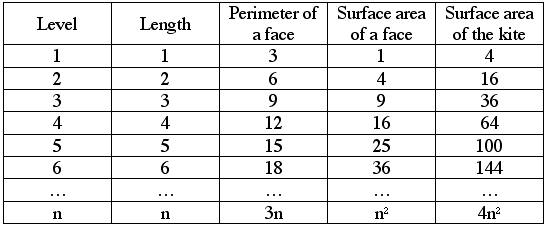When you move into volume, you get some very exciting mathematics that comes from these simple kits. I ask lots of questions and answer none. I have included a sample of my questioning. As you can see in the kites, level 1 has a volume of 1 tetrahedron. In level two, we should have a volume of 8 kites. Do we? We can count only four kites with a big hole in the center. What must the volume of that hole be? (Ans: 4 kites) What is the shape of that hole? (Ans: an octahedron). How do you know? (Here I place a straw octahedron inside the 2nd level kite and show that it does fill the space.) Do you believe that that octahedron has a volume of 4 kites? (Ans: most say No) I didn't either. This one (holding up the octahedron) does not seem four times the size of this one (holding up the single tetrahedron). Let's see, if the octahedron is really four kites, then half of it (a square pyramid is two kites. (Here I hold up a square pyramid made of straw and place it over the top of the octahedron.) And half of that (hold up a pyramid made by slicing the square pyramid from the vertex along the diagonal of the square base) must be one. Is it? Are you certain? Let's see. I can place the last one in the octahedron and see that it must be 1/4 of the octahedron. So if the octahedron is 4 kites, then this last must be one. Or vise versa, if the last one is one, then the octahedron must be 4 kites. Let's see if this last pyramid is one kite. (Here I place the last pyramid next to the single tetrahedron.) Do they look like they have the same volume? Let's see. Two of the faces are the congruent. Let's make them the bases. (As I rotate the last pyramid, I slide it up to the tetrahedron and we all notice that it matches up with the tetrahedron [has the same height].) Do they have the same volume? Why? (Ans: Same base and same height = same volume. This is a nice example of sheering and Cavalieri's Theorem.) Now, looking at the volume of each level, level 1 has volume 1 with 1 kite. Level 2 has volume 8 with four kites and one octahedron hole. But what about level 3? What should the volume be? (Ans: 27) Can you count the 27. How many kites can you see? (Ans: 10) How many holes? (Ans: 4) So we have 10 kites and 4 holes times four kites for each hole. That is 10 + 16 or 26. So the volume is 26, right? (Ans: NO) So where is the missing kite? Find if for me ... in the kites. Where is it? Describe it to me. (Ans: In the center of the three level kite but upside down. I call these the inverted or invisible kites.)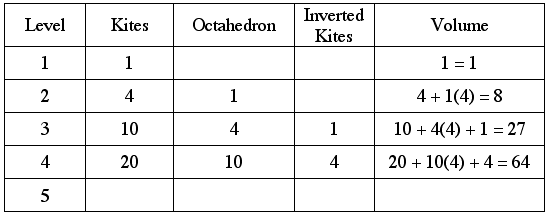What pattern are you starting to see? (If they don't see the tetrahedron/pyramidal numbers, then build the values by looking at the differences that are triangular numbers. Or just build the 4th and 5th level and then look for the pattern.)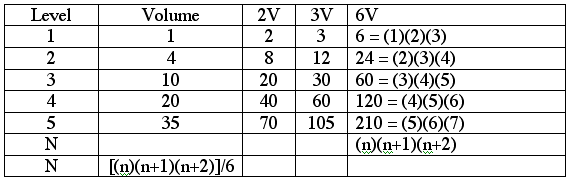So, placing this back into our table we get three different equations. The first if from what we just achieved. The last two are from this same result but shifted down one or two rows.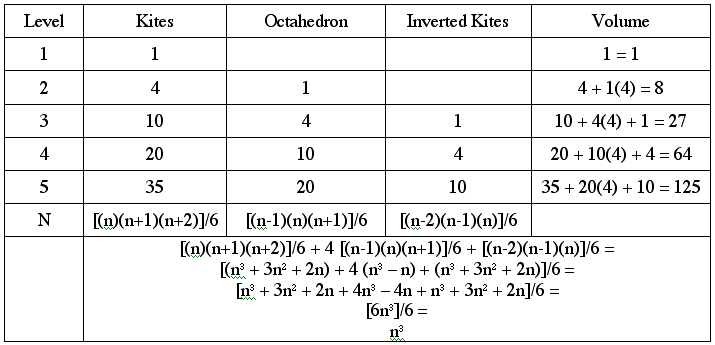Isn't that great?! Isn't it great how the volume as the sum of the three parts (tetrahedron, octahedrons, and inverted tetrahedrons) equals the volume as we know it (n3). This is sweet! (QED)PCMI@MathForum Home || IAS/PCMI Home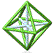© 2001 - 2018 Park City Mathematics Institute IAS/Park City Mathematics Institute is an outreach program of the School of Mathematics at the Institute for Advanced Study, Einstein Drive, Princeton, NJ 08540 Send questions or comments to: Suzanne Alejandre and Jim King